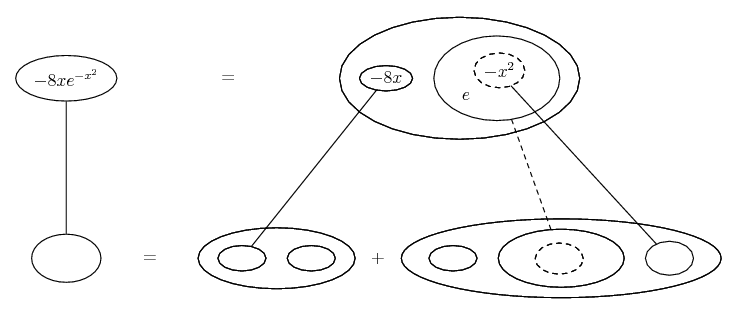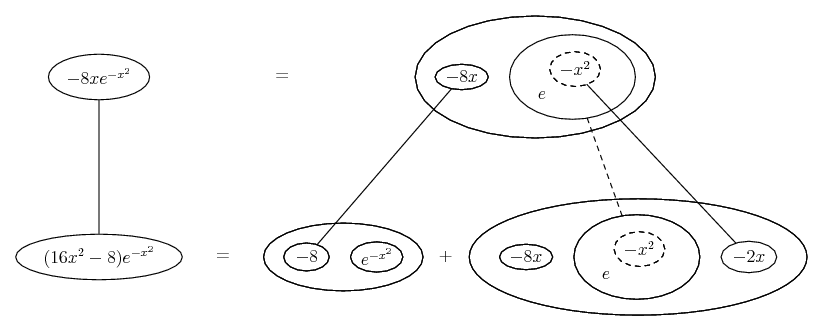# Thread: Second Derivative

1. ## Second Derivative

Hello, I have a question I am not sure how to fully solve.

F(x) = 4e^-x^2; Find F''(3)

so I take the first derivative which is = -8xe^-x^2
now to take the 2nd derivative I would use the product rule with -8x * e^-x^2 and I got 16xe^2e^(-x)^*2 * -8e^(-x)^2

clearly, this does not seem to be correct because if I plug in 3 it would be an extremely small/large number.

2. Just in case a picture helps...Spoiler:... where... is the chain rule, here wrapped in the legs un-crossed version of...... the product rule. Straight continuous lines differentiate downwards with respect to x, and the straight dashed line similarly but with respect to the dashed balloon expression (the inner function of the composite which is subject to the chain rule).
_________________________________________
Don't integrate - balloontegrate!

Balloon Calculus; standard integrals, derivatives and methods

Balloon Calculus Drawing with LaTeX and Asymptote!

3.Originally Posted by cokeclassicHello, I have a question I am not sure how to fully solve.

F(x) = 4e^-x^2; Find F''(3)

so I take the first derivative which is = -8xe^-x^2
now to take the 2nd derivative I would use the product rule with -8x * e^-x^2 and I got 16xe^2e^(-x)^*2 * -8e^(-x)^2

clearly, this does not seem to be correct because if I plug in 3 it would be an extremely small/large number.
You 2nd derivative is off just a little.

$\displaystyle (16x^2-8)*e^{x^{-2}}$

#### Search Tags

derivative